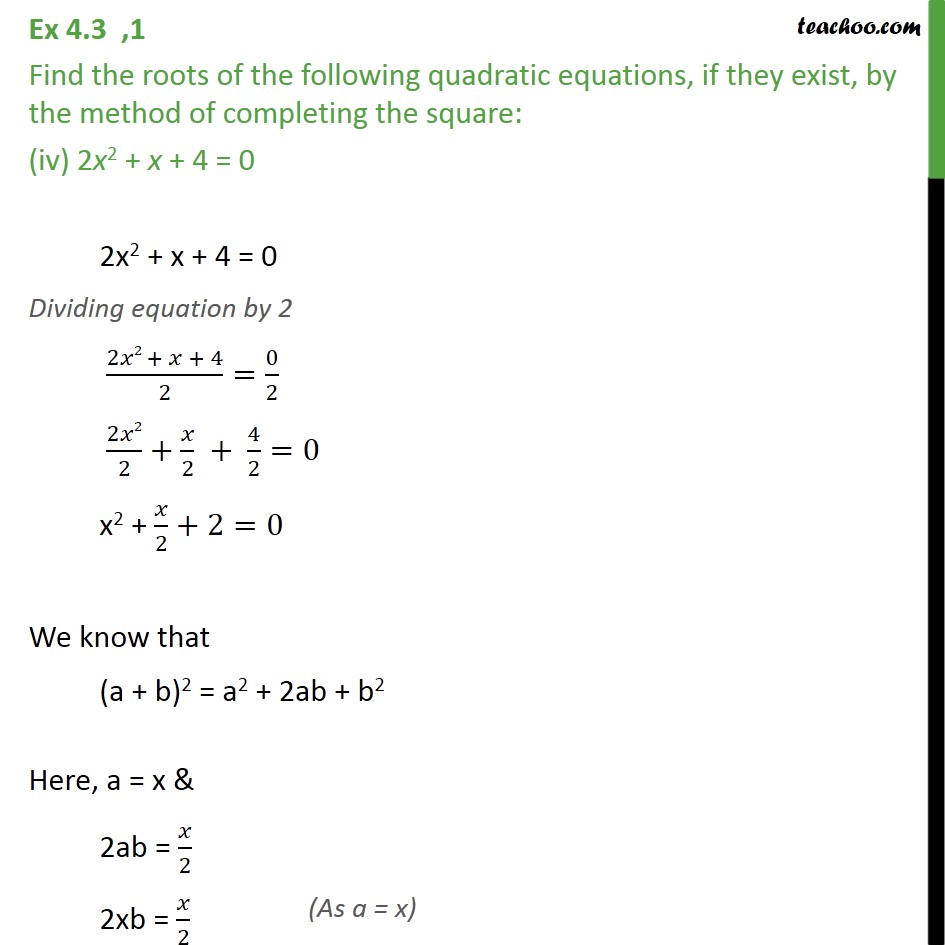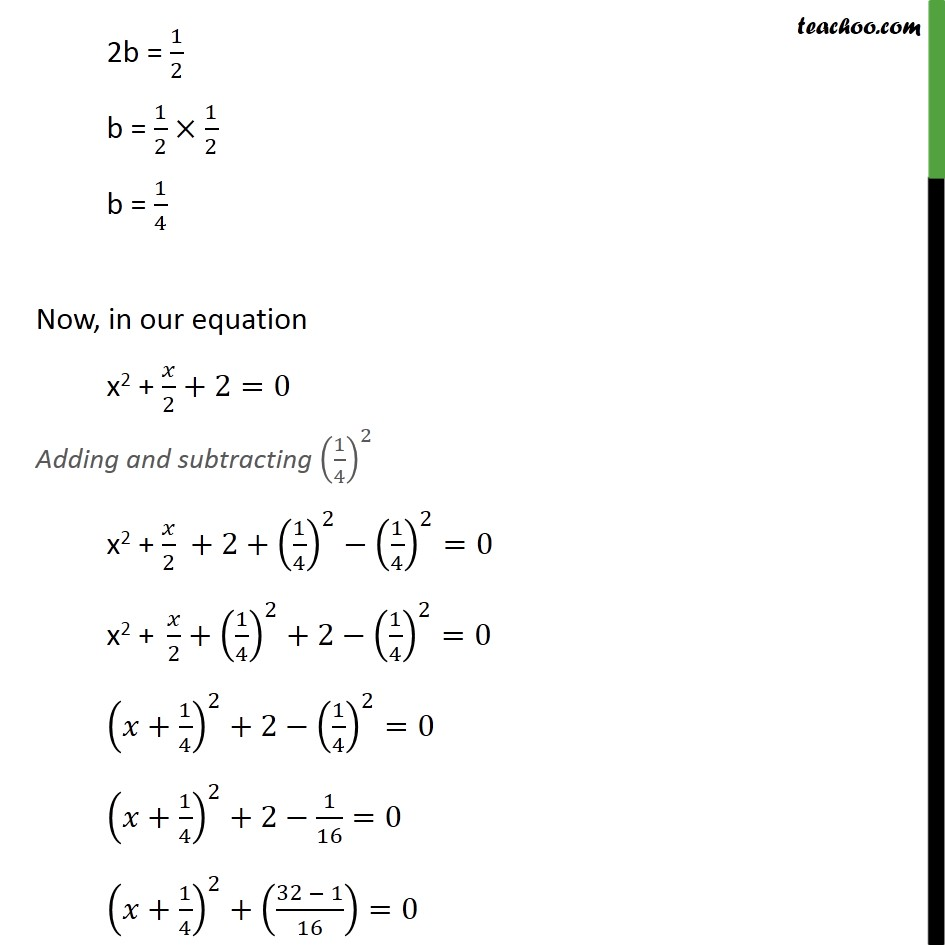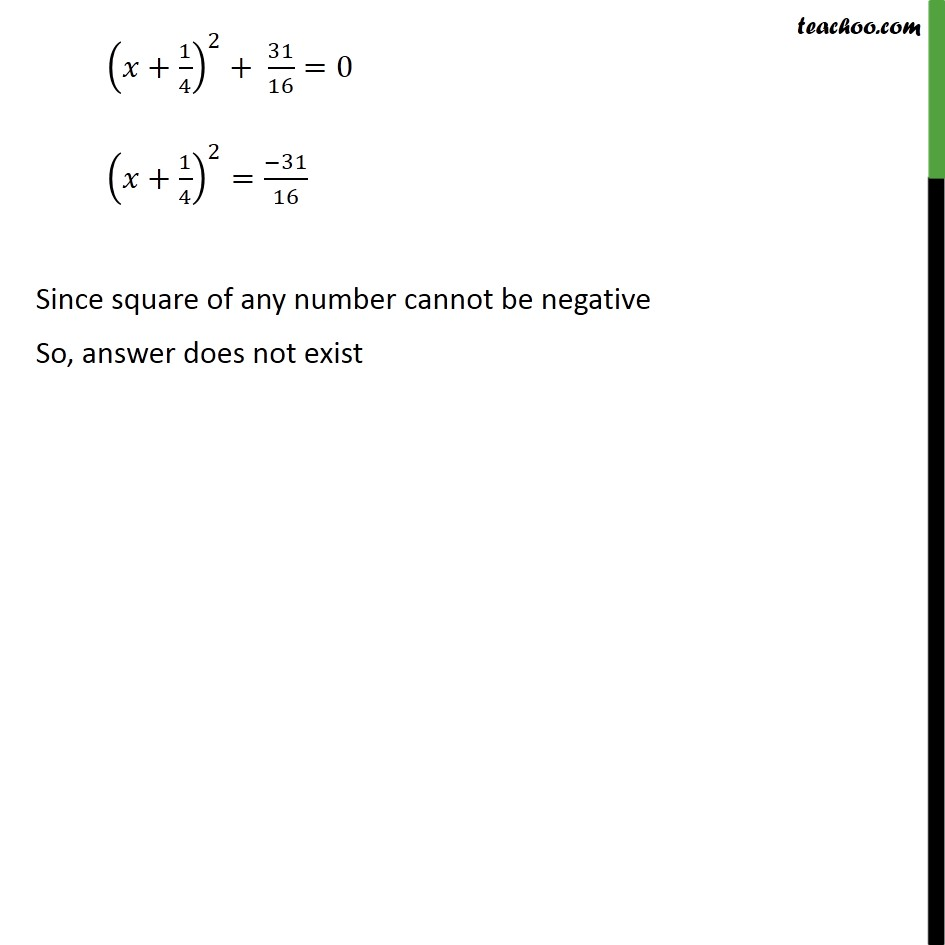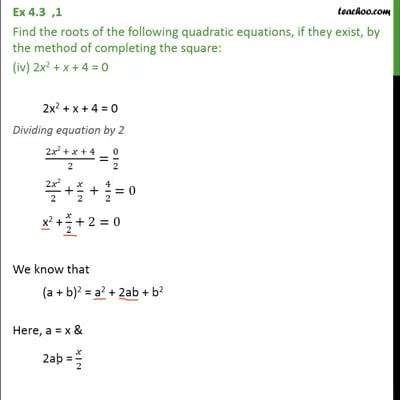Ex 4.3

Chapter 4 Class 10 Quadratic Equations (Term 2)
Serial order wiseThis video is only available for Teachoo black users

### Transcript

Ex 4.3 ,1 Find the roots of the following quadratic equations, if they exist, by the method of completing the square: (iv) 2x2 + x + 4 = 0 2x2 + x + 4 = 0 Dividing equation by 2 (2𝑥2 + 𝑥 + 4)/2=0/2 2𝑥2/2+𝑥/2 + 4/2=0 x2 + 𝑥/2+2=0 We know that (a + b)2 = a2 + 2ab + b2 Here, a = x & 2ab = 𝑥/2 2xb = 𝑥/2 2b = 1/2 b = 1/2×1/2 b = 1/4 Now, in our equation x2 + 𝑥/2+2=0 Adding and subtracting (1/4)^2 x2 + 𝑥/2 +2+(1/4)^2−(1/4)^2=0 "x2 + " 𝑥/2+(1/4)^2+2−(1/4)^2=0 (𝑥+1/4)^2+2−(1/4)^2=0 (𝑥+1/4)^2+2−1/16=0 (𝑥+1/4)^2+((32 − 1)/16)=0 (𝑥+1/4)^2+ 31/16=0 (𝑥+1/4)^2=(−31)/16 Since square of any number cannot be negative So, answer does not exist